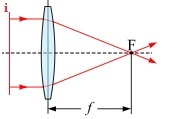# C Program for focal length of a lens

CServer Side ProgrammingProgramming

Given two floating values; image distance and object distance from a lens; the task is to print the focal length of the lens.

What is focal length?

Focal length of an optical system is the distance between the center of lens or curved mirror and its focus.

Let’s understand with the help of figure given below −In the above figure i is the object, and F is the image of the object which is formed and f is the focal length of the image.

So to find the focal length of the image from the lens the formula is −

1F= 1O+1I

Where, F is the focal length.

O is the total distance of the lens and the object.

I is the total distance between lens and the image formed by the lens.

## Example

Input: image_distance=5, object_distance=10
Output: Focal length of a lens is: 3.333333
Explanation: 1/5 + 1/10 = 3/10🡺 F = 10/3 = 3.33333333

Input: image_distance = 7, object_distance = 10
Output: Focal length of a lens is: 4.1176470

Approach we are using to solve the above problem

• Take the input of the image_disance and object_distance.
• Find the sum 1/image_distance and 1/object_distance and return the result divided by 1.
• Print the result.

## Algorithm

Start
Step 1-> In function float focal_length(float image_distance, float object_distance)
Return 1 / ((1 / image_distance) + (1 / object_distance))

Step 2-> In function int main()
Declare and initialize the first input image_distance = 5
Declare and initialize the second input object_distance = 10
Print the results obtained from calling the function focal_length(image_distance, object_distance)
Stop

## Example

Live Demo

#include <stdio.h>
// Function to find the focal length of a lens
float focal_length(float image_distance, float object_distance) {
return 1 / ((1 / image_distance) + (1 / object_distance));
}
// main function
int main() {
// distance between the lens and the image
float image_distance = 5;
// distance between the lens and the object
float object_distance = 10;
printf("Focal length of a lens is: %f\n", focal_length(image_distance, object_distance));
return 0;
}

## Output

Focal length of a lens is: 3.333333